# Digital Electronics - Boolean Algebra and Logic Simplification

### Exercise :: Boolean Algebra and Logic Simplification - General Questions

36.

Which statement below best describes a Karnaugh map?

 A. A Karnaugh map can be used to replace Boolean rules. B. The Karnaugh map eliminates the need for using NAND and NOR gates. C. Variable complements can be eliminated by using Karnaugh maps. D. Karnaugh maps provide a cookbook approach to simplifying Boolean expressions.

Answer: Option D

Explanation:

No answer description available for this question. Let us discuss.

37.

Applying DeMorgan's theorem to the expression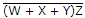, we get ________.

 A.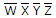B.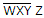C.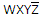D.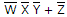Answer: Option D

Explanation:

No answer description available for this question. Let us discuss.

38.

Which of the examples below expresses the distributive law of Boolean algebra?

 A. (A + B) + C = A + (B + C) B. A(B + C) = AB + AC C. A + (B + C) = AB + AC D. A(BC) = (AB) + C

Answer: Option B

Explanation:

No answer description available for this question. Let us discuss.

39.

Applying DeMorgan's theorem to the expression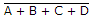, we get ________.

 A.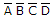B.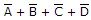C.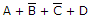D.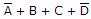Answer: Option A

Explanation:

No answer description available for this question. Let us discuss.

40.

Which of the following is an important feature of the sum-of-products (SOP) form of expression?

 A. All logic circuits are reduced to nothing more than simple AND and OR gates. B. The delay times are greatly reduced over other forms. C. No signal must pass through more than two gates, not including inverters. D. The maximum number of gates that any signal must pass through is reduced by a factor of two.

Answer: Option C

Explanation:

No answer description available for this question. Let us discuss.

#### Current Affairs 2021

Interview Questions and Answers